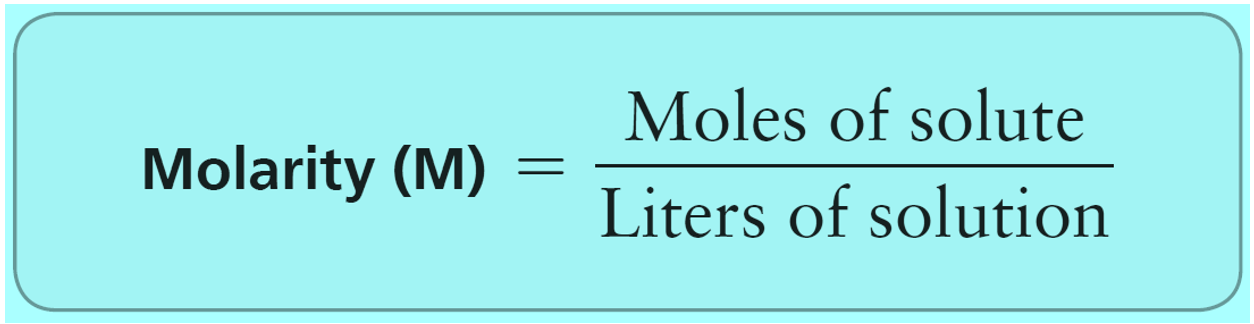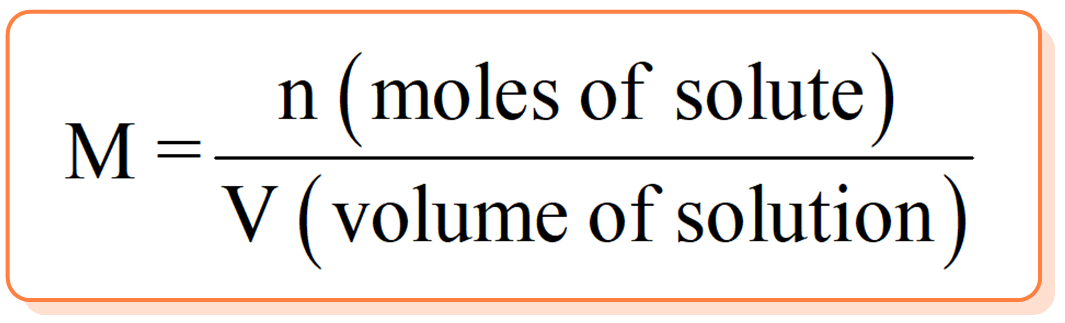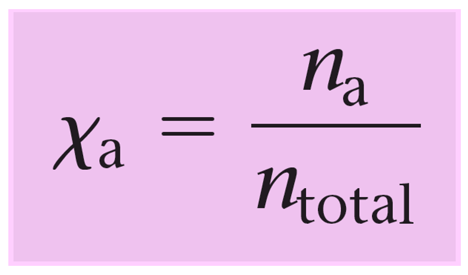## General Chemistry

The molality (m) is the number of moles of solute dissolved in 1000 g (1.00 kg) of solvent. Along with molarity, mass percent, and mole fraction, it is one of the common forms of concentration units. The formula for molality is:Notice that molality is defined with respect to kilograms solvent, not kilograms solution.

For example, what is the molality of a solution prepared by dissolving 26.3 g of ethylene glycol (C2H6O2) in 1.30 kg of water?

This is a straightforward problem, and the only conversion we need to do before using the numbers in the formula is to convert the grams to moles of ethylene glycol.

${\rm{n}}\,\left( {{{\rm{C}}_{\rm{2}}}{{\rm{H}}_{\rm{6}}}{{\rm{O}}_{\rm{2}}}} \right)\;{\rm{ = }}\;{\rm{26}}{\rm{.3}}\,{\rm{g}}\,{\rm{ \times }}\,\frac{{{\rm{1}}\,{\rm{mole}}}}{{{\rm{62}}{\rm{.1}}\,{\rm{g}}}}\;{\rm{ = }}\,{\rm{0}}{\rm{.4235}}\,{\rm{mol}}$

We will round off to three significant figures at the next step when the molality is calculated:

${\rm{m}}\,\left( {{{\rm{C}}_{\rm{2}}}{{\rm{H}}_{\rm{6}}}{{\rm{O}}_{\rm{2}}}} \right)\;{\rm{ = }}\;\frac{{{\rm{n}}\,\left( {{{\rm{C}}_{\rm{2}}}{{\rm{H}}_{\rm{6}}}{{\rm{O}}_{\rm{2}}}} \right)\,}}{{{\rm{kg}}\,{\rm{(}}{{\rm{H}}_{\rm{2}}}{\rm{O)}}}}\;{\rm{ = }}\,\frac{{{\rm{0}}{\rm{.4235}}\,{\rm{mol}}\,}}{{{\rm{1}}{\rm{.30}}\,{\rm{kg}}}}{\rm{ = }}\,{\rm{0}}{\rm{.326}}\,{\rm{mol/kg}}$

Let’s see what the main difference is between molality compared to molarity. In molarity, we have the ratio of the moles of solute to the volume of the solution.So, when asked to prepare a solution expressed in molarity, we weigh the solute and add as much solvent as needed to reach the needed volume.

Although this does not usually make a significant difference, the disadvantage of using molarity is that it is temperature-dependent because the volume can change with temperature. On the other hand, molality is temperature-independent since we are only using the mass of the solute and the solvent.

The concentration of antifreeze solution, for example, is always calculated in molality since the molarity won’t be as accurate in high-temperature fluctuations. The change of freezing and boiling point of solutions will be discussed later in the colligative properties.

You should know, however, that molarity and other forms of concentration are correlated with molality, and one can be calculated using the data for others.

For example, Ethylene glycol (C2H6O2) is used in automobile antifreeze as a 40.0 mass % aqueous solution. Calculate the molarity, molality, and mole fraction of the ethylene glycol if the density of the solution is 1.05 g/cm3.

If the quantity of the solution is not given, you can assume any number. Let’s set it to a 1.00 L solution this time. That is 1000 mL or 1000 cm3.

The molarity of ethylene glycol is M = n/V. We have the volume, so next is to find the moles by determining the mass first.

The mass of the solution will be:

${\rm{m}}\,{\rm{ = d}}\,{\rm{ \times }}\,{\rm{V}}\,{\rm{ = }}\,{\rm{1}}{\rm{.05}}\;\frac{{\rm{g}}}{{\cancel{{{\rm{c}}{{\rm{m}}^{{\rm{3\;}}}}}}}}\,{\rm{ \times }}\,{\rm{1000}}\;\cancel{{{\rm{c}}{{\rm{m}}^{\rm{3}}}}}{\rm{\; = 1050 g}}$

In this solution, we have 40.0 % ethylene glycol which is calculated as follows:

m (C2H6O2) = m (solution) x 0.400 = 1050 g x 0.4 = 420. g

The moles of ethylene glycol is found by the ratio of the mass over the molar mass:

${\rm{n}}\,\left( {{{\rm{C}}_{\rm{2}}}{{\rm{H}}_{\rm{6}}}{{\rm{O}}_{\rm{2}}}} \right)\;{\rm{ = }}\;{\rm{420}}{\rm{.}}\,{\rm{g}}\,{\rm{ \times }}\,\frac{{{\rm{1}}\,{\rm{mole}}}}{{{\rm{62}}{\rm{.1}}\,{\rm{g}}}}\;{\rm{ = }}\,{\rm{6}}{\rm{.76}}\,{\rm{mol}}$

The molarity of ethylene glycol is:

${\rm{M}}\,\left( {{{\rm{C}}_{\rm{2}}}{{\rm{H}}_{\rm{6}}}{{\rm{O}}_{\rm{2}}}} \right)\;{\rm{ = }}\;\frac{{\rm{n}}}{{\rm{V}}}\;{\rm{ = }}\,\frac{{{\rm{6}}{\rm{.76}}\,{\rm{mol}}}}{{{\rm{1}}{\rm{.00}}\,{\rm{L}}}}\;{\rm{ = }}\,{\rm{6}}{\rm{.76}}\,{\rm{mol/L}}$

To find the molality of ethylene glycol, we need to first calculate the mass of the water.

The mass of water (the solvent) is equal to the mass (solution) – mass (C2H6O2) = 1050 g – 420. g = 630. g = 0.630 kg

Therefore, the molality of ethylene glycol is:

${\rm{m}}\,\left( {{{\rm{C}}_{\rm{2}}}{{\rm{H}}_{\rm{6}}}{{\rm{O}}_{\rm{2}}}} \right)\;{\rm{ = }}\;\frac{{{\rm{n}}\,\left( {{{\rm{C}}_{\rm{2}}}{{\rm{H}}_{\rm{6}}}{{\rm{O}}_{\rm{2}}}} \right)\,}}{{{\rm{kg}}\,{\rm{(}}{{\rm{H}}_{\rm{2}}}{\rm{O)}}}}\;{\rm{ = }}\,\frac{{{\rm{6}}{\rm{.76}}\,{\rm{mol}}\,}}{{{\rm{0}}{\rm{.630}}\,{\rm{kg}}}}{\rm{ = }}\,{\rm{10}}{\rm{.7}}\,{\rm{mol/kg}}$

The mole fraction is the ratio of the moles of ethylene glycol over the moles of the solution (total moles of C2H6Oand H2O).$X\,{\rm{(}}{{\rm{C}}_{\rm{2}}}{{\rm{H}}_{\rm{6}}}{{\rm{O}}_{\rm{2}}}{\rm{)}}\;{\rm{ = }}\,\frac{{{\rm{n}}\,{\rm{(}}{{\rm{C}}_{\rm{2}}}{{\rm{H}}_{\rm{6}}}{{\rm{O}}_{\rm{2}}}{\rm{)}}\;}}{{{\rm{n}}\,{\rm{(}}{{\rm{C}}_{\rm{2}}}{{\rm{H}}_{\rm{6}}}{{\rm{O}}_{\rm{2}}}{\rm{)}}\;{\rm{ + }}\,{\rm{n}}\,{\rm{(}}{{\rm{H}}_{\rm{2}}}{\rm{O)}}}}$

Next, we calculate the moles of water:

${\rm{n}}\,\left( {{{\rm{H}}_{\rm{2}}}{\rm{O}}} \right)\;{\rm{ = }}\;{\rm{630}}{\rm{.}}\,{\rm{g}}\,{\rm{ \times }}\,\frac{{{\rm{1}}\,{\rm{mole}}}}{{{\rm{18}}{\rm{.0}}\,{\rm{g}}}}\;{\rm{ = }}\,{\rm{35}}{\rm{.0}}\,{\rm{mol}}$

Therefore, the total moles = 6.76 mol + 35.0 = 41.8 mol, and we can now use it to calculate the mole fraction of ethylene glycol:

$X\,{\rm{(}}{{\rm{C}}_{\rm{2}}}{{\rm{H}}_{\rm{6}}}{{\rm{O}}_{\rm{2}}}{\rm{)}}\;{\rm{ = }}\,\frac{{{\rm{6}}{\rm{.76}}\,{\rm{mol}}\;}}{{{\rm{6}}{\rm{.76}}\,{\rm{mol}}\;{\rm{ + }}\,{\rm{35}}{\rm{.0}}\,{\rm{mol}}}}\; = \;0.162$

Check Also